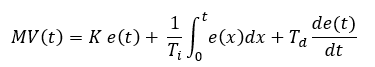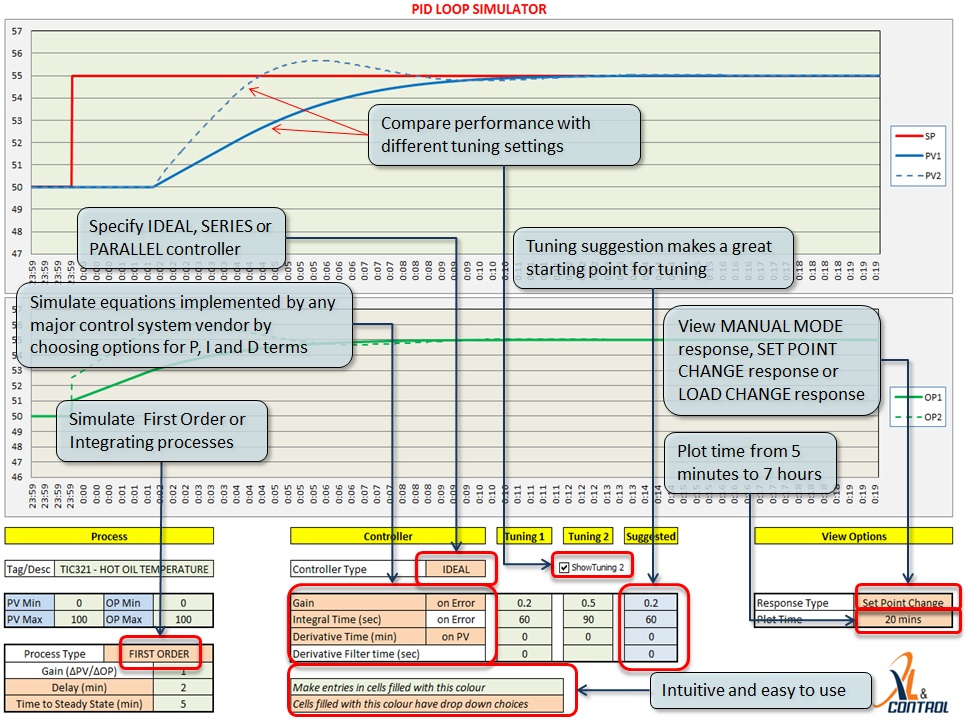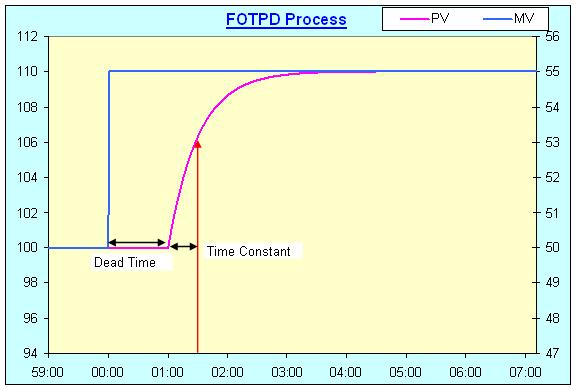# PID Loop Simulator

Description

The PID Loop Simulator is an Excel tool to simulate a Proportional, Integral and Derivative (PID) controller on a First Order Time Delay (FOTPD) process. Both open and closed loop processes can be simulated using this powerful tool. This is a great tool for learning the basics of PID control and loop tuning..xlsx file (92 KB)

## How to use

Enter the process parameters into the “Process” box. The First Order Time Delay (FOTPD) process has 3 parameters – Process Gain, Lag Time and Delay Time. Details of these parameters are given in the "Process Model" section below.

Choose the “Open Loop” option and the loop drawing changes to the open loop configuration. In this mode, the controller is not used.The chart shows the open loop response. The Manipulated Value is plotted on the secondary axis.To simulate the closed loop response, key the controller parameters into the “Controller” box. These parameters are the controller gain, integral time and the derivate time. Choose the “Closed Loop” option to view the closed loop response on the chart.

This tool can be used to study the effect of various controller parameters on the closed loop behaviour and to study techniques such as Ziegler Nichols method of controller tuning.

The tool simulates the parallel form of the PID equation, this is form that is widely used in academic environments. The equation implemented is:where

t = Time
MV = Manipulated value
K = Controller Gain
e = Controller error = Set Point - Process Value
Ti = Integral Time
Td = Derivative Time

## Feedback and Contributions from Visitors

The PID Simulator tool has been the most downloaded tool on this site and I am grateful to the users who sent me a lot of positive feedback about it.

Torcuato Fernandez pointed out an error in original version, which has since has been corrected. I would like to thank Torcuato for pointing out the error.

I would also like to thank Hannu Lehmuskuja for pointing out an error related to the delay.

Jose Maria, has shared some of his work with me and has kindly agreed to let me share it with others through this site. He has added scrollbars to the tool, which assist the user understand how the controller settings affect the loop.

For any comments and discussions on this app, you can join and initiate a discussion on the open Linked-in group Engineering Using Spreadsheets.
Alternatively, email mehta.nitin@gmail.com

I look forward to getting more great suggestions and comments. Thank you!

## PID Loop Simulation and Tuning tool for use in Industry

The use of PID Controllers is widespread in the process industry. Industrial controllers use different forms of the PID controllers. A powerful PID simulation tool that can simulate these different forms and be used for loop tuning is available at the business site www.xlncontrol.com. A free demo version is also available, the demo version simulates the ideal verion of the PID controller.

The features of this tool are shown in the image below.## Process Model

To use the simulator, we need a model of the process. Obtaining the process parameters is known as System Identification.

Most chemical processes fall into one of 2 categories - first order process with dead time (FOPDT) and integrating processes with dead time. This app works with the former. (A simulation of a PI controller on a delay free integrating process is also available on this site, see PI Control of Integrating Process).

An FOPDT process is characterised by 3 parameters:

1. Process Gain - the ratio of the change in process variable to the ratio of the change in manipulated variable

2. Time constant - which measures the speed of response

3. Dead time - time between moving the manipulated variable and start of the process response

One of the most common ways of obtaining these parameters is by doing a step test. To do this, wait for the process to be steady and then step the Manipulated Variable (MV). The process variable (PV) will move as shown below.Calculate the parameters as follows:

Dimensionless Gain = (Change in PV/PV range)/(Change in MV/MV Range)

Time constant = Time taken for the PV to change by 63.2% of the final change

Dead time = Time for the PV to start moving after the change in the MV

For the step response shown in the figure above, Dimensionless Gain = (10/200)/(5/100), where PV range = 200 units and MV range = 100 units

Time constant = 30 sec

Key in these parameters into the simulator and study the effects of changing the tuning parameters on the response of the system.

## Why 63.2% ?

The response of a delay free first order system is described by:

Change in PV = Process gain x (1 - exp(-Time/Time Constant))x Change in MV

Since the final change in PV = Process gain x Change in MV, this equation can be written as

Change in PV = Final Change in PV x (1 - exp(-Time/Time Constant))

At time = time constant,

Change in PV = (1 - exp(-1)) x Final Change in PV = 0.632 x Final Change in PV or 63.2% of the final change in the PV

I would like to thank Hannu Lehmuskuja to correcting an error in these equations.

## System Idenfitication Tool

In the industrial environment, it is often difficult to obtain process models due to noisy data. A powerful  for system identfication is available at the business site www.xlncontrol.com

## How it works

There are 2 worksheets in the Excel file, the calculations required for the simulation are done on the sheet "PID Calculations".

Defined Formulae are used in the computation.

The first order process is calculated using a difference equation, given by:

Process Value = b x (Output at time offset by delay time) - a x Last PV; where

a = - exp ( -1 / Process Time Constant) and

b = Process Gain x (1 + a)

(Thanks to Hannu Lehmuskuja for pointing out an error in the documentation on this page.)

The error is calculated by taking the difference between the PV and SP values. The error values are accumulated in column F for the integral term calculation and the derivative term is calculated by taking the difference between the current error and the last error. The PID calculation determines the next output value. The PID calculation is:

The next output value is calculated using:

Controller Gain x error + Accumulated error / Integral time + Derivative x (Current error - Previous error)

The calculations are repeated at every second to obtain the response, which is plotted on the chart on the first sheet.